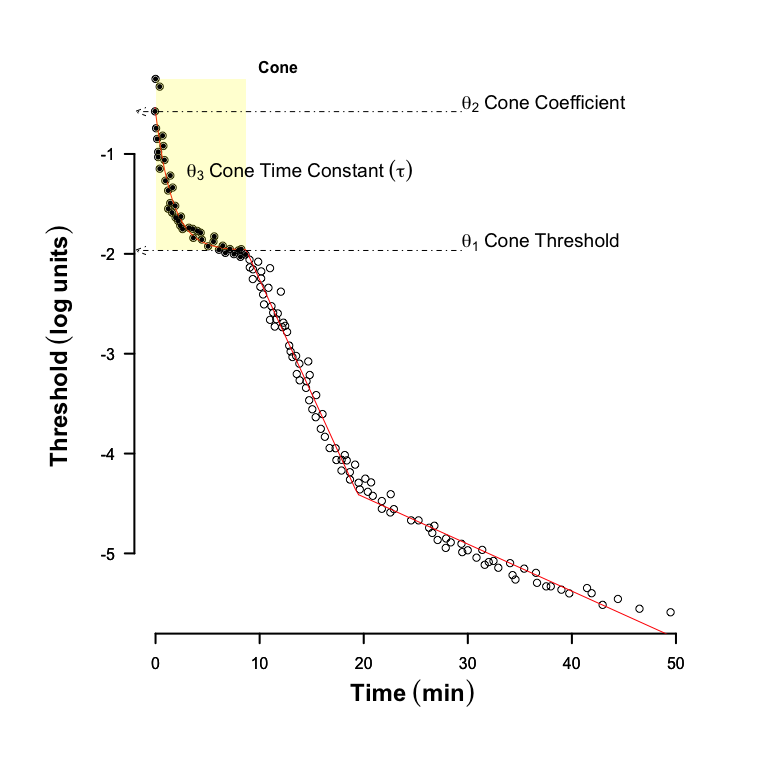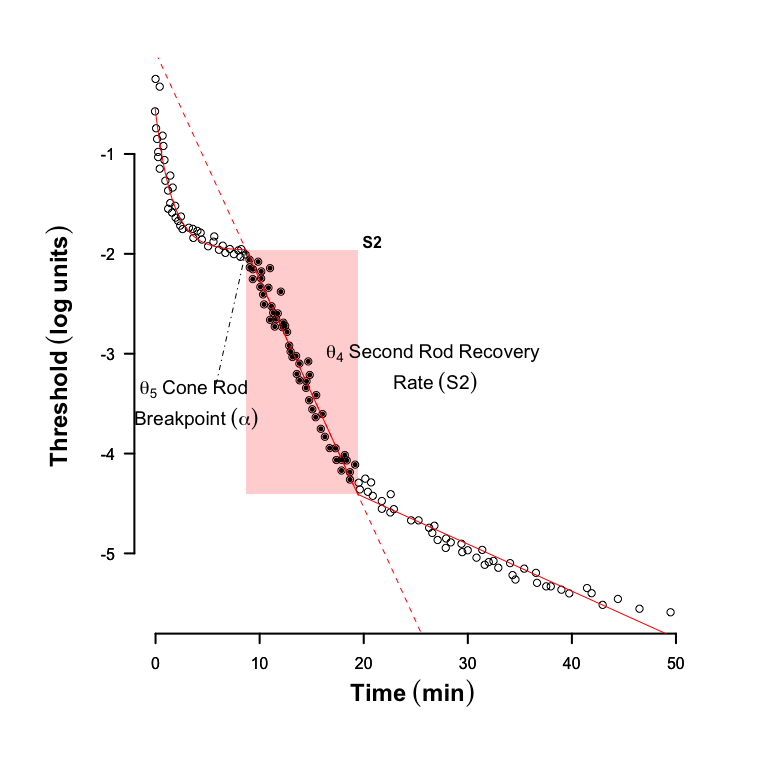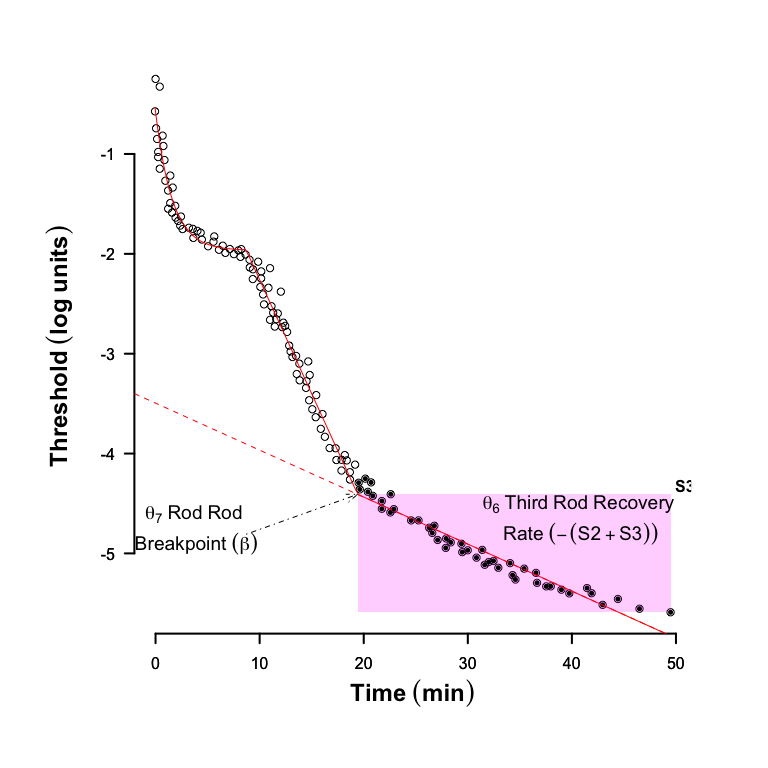# The Parameters of a Dark Adaptation Model Explained

#### 2016-06-02

The following plots explain the parameters of the model used to predict thresholds ($$Thrs$$) following a photo-bleach which transforms more than 30% of the retinal photopigment. These data were extracted from Figure 1 in Pugh 1975.

There are typically three phases to the recovery of visual sensitivity in dark adaptation data; an early cone phase, the second rod phase sometimes called S2, and a final rod phase or S3 and are shown in the figure below.

The initial phase depicts the cone response ($$Thrs_c$$), and can be represented by three parameters; the absolute cone threshold ($$\theta_1$$, log$$_{10}$$(cd.m$$^{\text{-}2}$$) scotopic), the cone coefficient ($$\theta_2$$, log$$_{10}$$(cd.m$$^{\text{-}2}$$) scotopic), and the time constant of cone recovery, sometimes known as tau ($$\theta_3$$, min).

$Thrs_c = \theta_1 + \theta_2.\exp\left(\frac{\text{-}t}{\theta_3}\right) .$

The cone coefficient can be thought of as the cone threshold at the instant following the flash, but as this variable can take non-physiological values it is best perhaps thought of a free variable that aids the fitting process.After the cone mediated phase there follows the rod phase, S2, which represents the rate limited restoration of photopigment and is defined by its slope, ($$\theta_4$$, log$$_{10}$$(cd.m$$^{\text{-}2}$$).min$$^{\text{-}1}$$) and the rod-cone transition time or alpha point ($$\theta_5$$, min). It is clear from the figure that the alpha point is defined by the slope and the intercept of the S2 phase.

The linear (on a log-lin plot) rod phase S2 is described in detail in Lamb and Pugh 2004.Similarly the next rod phase, is described by the sum of the slopes of S2 and S3 ($$\theta_6$$, log$$_{10}$$(cd.m$$^{\text{-}2}$$).min$$^{\text{-}1}$$) and the rod-rod transition time, occasionally referred to as the beta point ($$\theta_7$$, min).

The final phase of rod recovery S3 may not be found in all subjects, e.g. a subject with reduced rod function may take an hour or more to reach this final phase.

##
## Attaching package: 'Dark'
## The following objects are masked _by_ '.GlobalEnv':
##
##     H, P7cThe model uses the transition times to control switch functions and hence allow each phase to combine.

The response threshold $$Thrs$$ can therefore be summarized as follows: $Thrs = Thrs_c + \underbrace{ \theta_4.h(t,\theta_5) }_{\mbox{S2}}+\underbrace{ \theta_6.h(t,\theta_7) }_{\mbox{S3}}$ where $$h(t,\theta_i)$$ is a switch function: $h(t,\theta_i) \begin{cases} 0 & \text{if t-\theta_i\leq0,} \\ t-\theta_i &\text{if t- \theta_i> 0.} \end{cases} \qquad i \in (5,7).$

The models in this package use a logistic function $h(t,\theta_i)\rightarrow \frac{1}{1+exp(-k(t-\theta_i))}.(t-\theta_i)\qquad i \in (5,7).$

This continuous switch function is computationally more efficient and allows an analytic approach to estimates of parameter variance.

Notice that since the threshold data are in log units, when plotted against time the apparently linear phases S2 and S3 describe exponential processes.